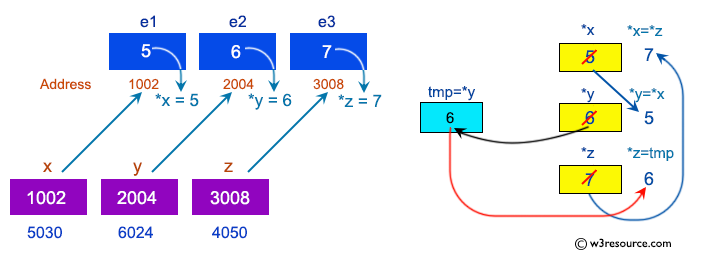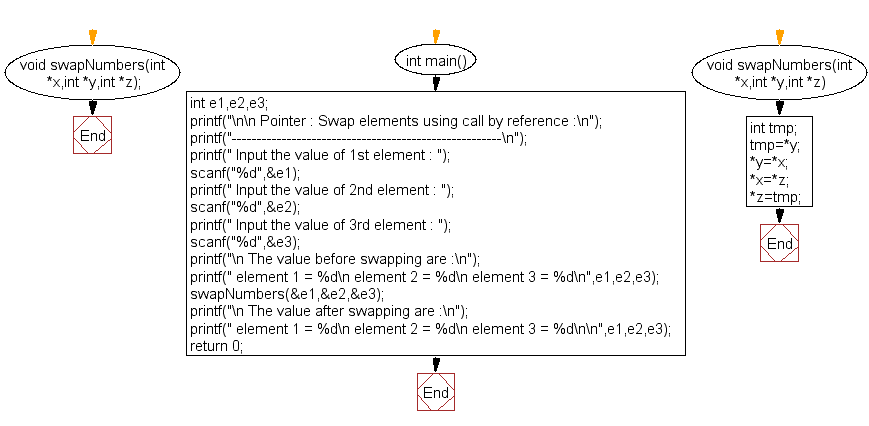﻿ C Program: Swap elements using call by reference - w3resource# C Exercises: Swap elements using call by reference

## C Pointer : Exercise-11 with Solution

Write a program in C to swap elements using call by reference.

Pictorial Presentation:Sample Solution:

C Code:

``````#include <stdio.h>
void swapNumbers(int *x,int *y,int *z);
int main()
{
int e1,e2,e3;
printf("\n\n Pointer : Swap elements using call by reference :\n");
printf("------------------------------------------------------\n");
printf(" Input the value of 1st element : ");
scanf("%d",&e1);
printf(" Input the value of 2nd element : ");
scanf("%d",&e2);
printf(" Input the value of 3rd element : ");
scanf("%d",&e3);

printf("\n The value before swapping are :\n");
printf(" element 1 = %d\n element 2 = %d\n element 3 = %d\n",e1,e2,e3);
swapNumbers(&e1,&e2,&e3);
printf("\n The value after swapping are :\n");
printf(" element 1 = %d\n element 2 = %d\n element 3 = %d\n\n",e1,e2,e3);
return 0;
}
void swapNumbers(int *x,int *y,int *z)
{
int tmp;
tmp=*y;
*y=*x;
*x=*z;
*z=tmp;
}
```
```

Sample Output:

``` Pointer : Swap elements using call by reference :
------------------------------------------------------
Input the value of 1st element : 5
Input the value of 2nd element : 6
Input the value of 3rd element : 7

The value before swapping are :
element 1 = 5
element 2 = 6
element 3 = 7

The value after swapping are :
element 1 = 7
element 2 = 5
element 3 = 6
```

Flowchart:C Programming Code Editor:

Have another way to solve this solution? Contribute your code (and comments) through Disqus.

What is the difficulty level of this exercise?

Test your Programming skills with w3resource's quiz.

﻿

## C Programming: Tips of the Day

How to format strings using printf() to get equal length in the output?

You can specify a width on string fields, e.g.

`printf("%-20s", "initialization..."); `

And then whatever's printed with that field will be blank-padded to the width you indicate.

The - left-justifies your text in that field.

Ref : https://bit.ly/34DMOc3